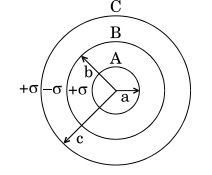# Three concentric metallic shells A, B and C of radii a,b and c (a<b<c) have surface charge densities  respectively as shown.(a) Obtain the expressions for the potential of three shells A, B and C.(b) If shells A and C are at the same potential, obtain the relation between a, b and c.(a) We know,

The potential of sphere 'A'  is given as,

Where,

then,

Such that, , then

Similarly,

Hence,

Similarly, the potential of sphere 'B'  is given as;

Such that, , then

Similarly,

Similarly for

(b) we know,

then, the given condition if shell A and C are equal;

On putting the values, we get

such that

c =

- this is the obtained relation between a, b and c.

## Related Chapters

### Preparation Products

##### Knockout NEET Sept 2020

An exhaustive E-learning program for the complete preparation of NEET..

₹ 15999/- ₹ 6999/-
##### Rank Booster NEET 2020

This course will help student to be better prepared and study in the right direction for NEET..

₹ 9999/- ₹ 4999/-
##### Knockout JEE Main Sept 2020

An exhaustive E-learning program for the complete preparation of JEE Main..

₹ 12999/- ₹ 6999/-
##### Test Series NEET Sept 2020

Take chapter-wise, subject-wise and Complete syllabus mock tests and get in depth analysis of your test..

₹ 4999/- ₹ 2999/-